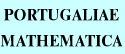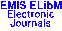PORTUGALIAEMATHEMATICA Vol. 56, No. 3, pp. 273-280 (1999)

Previous Article

Next Article

Contents of this Issue

Other Issues

ELibM Journals

ELibM Home

EMIS Home

## Characterization for Relations on Some Summability Methods

### W.T. Sulaiman

P.O. Box 120054,
Doher - QATAR

Abstract: In this paper we characterize a previous result proved by us connecting the summability methods $|\overline N,p_{n}|_{k}$ with either $|N,q_{n}|_{k}$ or $|\overline N,w_{n}|_{k}$ for given sequences $\{p_{n}\}$, $\{q_{n}\}$ and $\{w_{n}\}$ of positive real constants. Other results are also deduced.

Full text of the article: